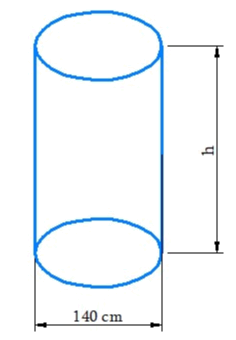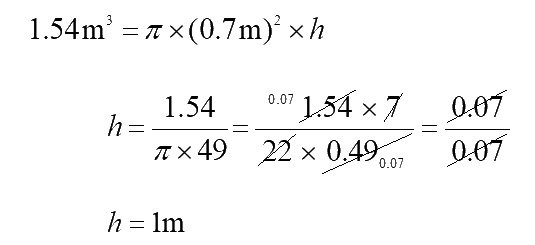In the verge of coronavirus pandemic, we are providing FREE access to our entire Online Curriculum to ensure Learning Doesn't STOP!

# Ex.11.4 Q5 Mensuration Solution - NCERT Maths Class 8

Go back to  'Ex.11.4'

## Question

Find the height of the cylinder whose volume is $$1.54{{\rm{m}}^3}$$ and diameter of the base is $$140\rm\, cm\,?$$

Video Solution
Mensuration
Ex 11.4 | Question 5

## Text Solution

What is Known?

Volume of the cylinder and diameter of the base of the cylinder is given.

What is unknown?
Height of the cylinder.

Steps:If the height of the cylinder $$= h$$

\begin{align}(r) = \frac{{140}}{2}\,{\rm{cm}} = 70\,{\rm{cm}}=0.7 \rm\,m\end{align}

Volume of the cylinder $$= \pi {r^2}h$$Thus, height of the cylinder is $$1\rm\,m.$$

Learn from the best math teachers and top your exams

• Live one on one classroom and doubt clearing
• Practice worksheets in and after class for conceptual clarity
• Personalized curriculum to keep up with school